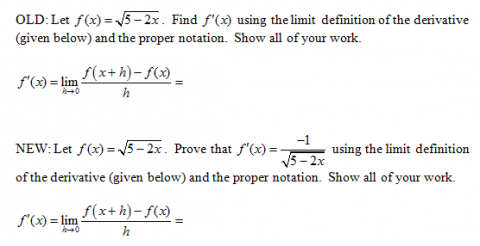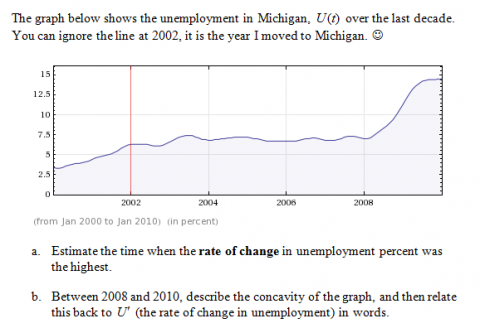I let my students use Wolfram Alpha when they are in class and when they are doing their homework (um, how would I stop them?).  Because of this, I’ve had to shift how I assess on more formal assignments.  For the record, it’s the same adjustment you might make if you were using ANY kind of Computer Algebra System (CAS).

The simplest shift is to stop asking for the answers to problems, and just give the students the answers.  After all, they live in a world where they CAN easily get the answers, so why pretend that it’s the answers that are important?  It’s the mathematical thinking that’s important, right?  Giving the students the answers turns problems into “proofs” where the evaluation (grade) is based on the thought-process to get from start to finish. It also eliminates the debate about whether to award points for a correct answer with no correct process.

Here are two examples of problems from a recent Calculus exam (old and new wording).I wish I had thought to do this years ago, because students who insist on just doing the “shortcut” (and not learning what limits are all about) now have nothing to show for themselves (the answer, after all, is right THERE).Again, a student that knows the derivative rules might get the right answer, but the right answer is now worth zero points.  The assessment is now clearly focused on the mathematical thinking using limits.

Another reason that I really like this is that it allows students to find mistakes that they are capable of finding “in the real world” where they can quickly use technology to get an answer.  They are now graded solely on their ability to explain, mathematically, the insides of a mathematical process.

Wolfram Alpha also allows me to pull real-world data into my tests much faster.  Here’s a question about curve shape (the graph is just a copy/paste from W|A):If you haven’t begun to think about how assessment should change in a world with ubiquitous and free CAS, you should.  You don’t have to change all your problems, but I think some of them should change.  Otherwise, we’re just testing students on the same thing that a computer does, and that doesn’t sit well with me.  If you can be replaced by a computer, you’re likely to be replaced by a computer.  Let’s make sure we’re teaching students how to think mathematically, not how to compute mathematically.

Possibly Related Posts: